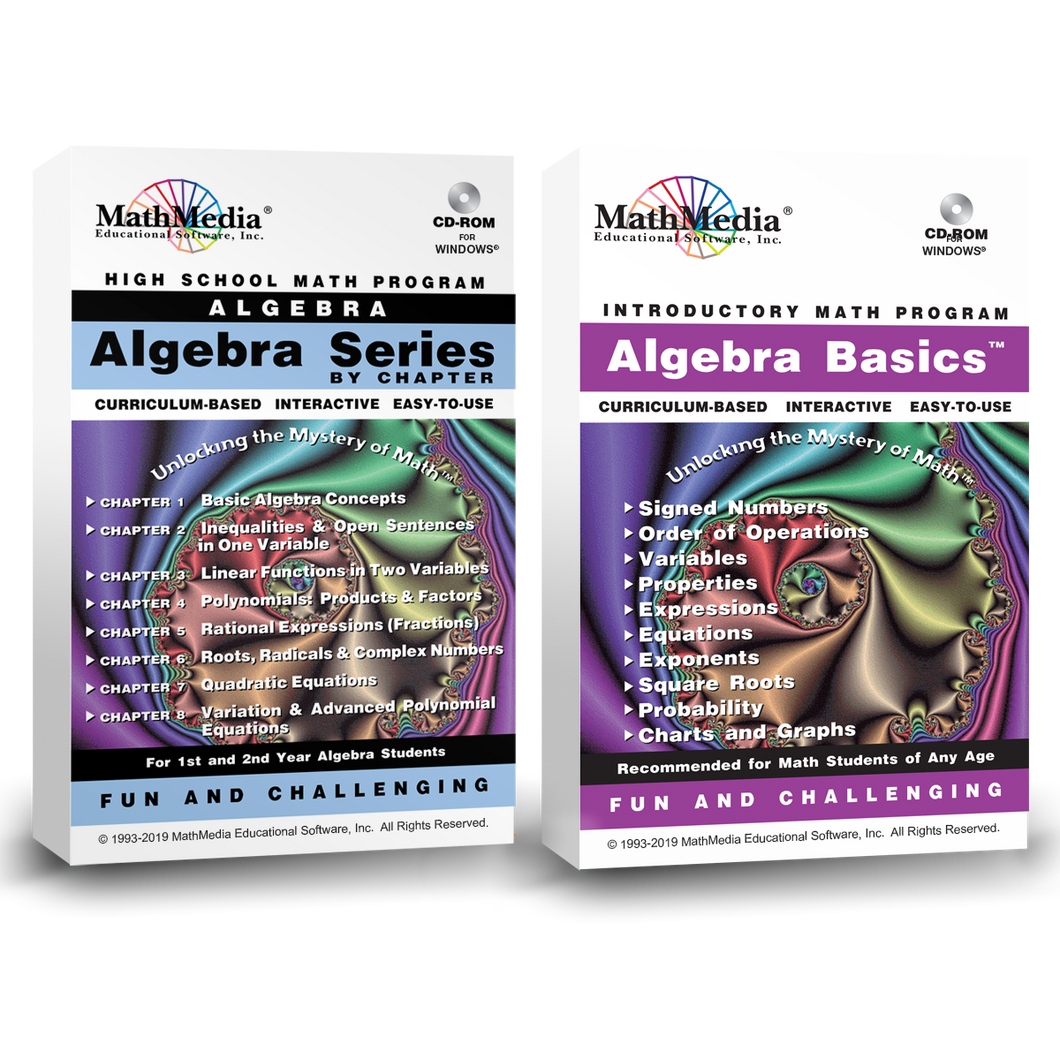# Algebra Series By Chapter + Pre-Algebra

Regular price \$309.00 \$77.25 Sale

Algebra By Chapter (8 Chapters) + Pre-Algebra
Set of 9 Programs

Algebra Basics (Pre-Algebra) includes:

Signed Numbers, Order of Operations, Variables, Properties, Expressions, Equations, Exponents, Square Roots, Sets, Probability, and Charts and Graphs. Pre-Algebra is the foundation for success in future math classes. MathMedia provides basic algebra lessons and practice for the beginning algebra student. Short quizzes are available for assessing each topic.

Begin learning algebra with "Algebra Basics" (Pre Algebra). The pre-requisite for learning algebra is to have a firm foundation in pre-algebra. Follow the study of pre algebra with eight (8) chapters (programs) of first year algebra software.

Algebra Series By Chapter includes
8 Chapters:

First Semester: Chapters 1 - 4
CH1: Basic Algebra Concepts
CH2: Inequalities & Open Sentences
CH3: Linear Functions
CH4: Polynomials - Products & Factors

Second Semester: Chapters 5 - 8
CH5: Rational Expressions (Algebraic Fractions)
CH6: Roots, Radicals & Complex Numbers
Algebra Series Curriculum SyllabusAlgebra Series Learning System